The availability of ICSE Class 9 Maths Solutions S Chand Chapter 13 Circle Ex 13(A) encourages students to tackle difficult exercises.

## S Chand Class 9 ICSE Maths Solutions Chapter 13 Circle Ex 13(A)

Question 1.
Calculate the length of a chord which is at a distance of 12 cm from the centre of a circle of radius 13 cm.
Solution:
In the given circle radius OA = 13 cm and ⊥ OM = 12 cm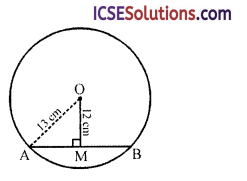Let length of chord AB = 2x
∵ OM ⊥ AB
∴ M is midpoint of AB
∴ AM = $$\frac { 2x }{ 2 }$$ = x
Now in right angled ∆OAM,
OA² = OM² + AM² (Pythagoras Theorem)
⇒ (13)² = (12)² + x²
⇒ 169 = 144 + x²
⇒ x² = 169 – 144
⇒ x² = 25 = (5)²
∴ x = 5
Length of chord AB = 2 x 5 = 10 cm

Question 2.
In the figure, the radius of the given circle, with centre C, is 6 cm, if the chord AB is 3 cm away from the centre, calculate its length.Solution:
In the given circle,
Radius CA = 6 cm and ⊥ CM = 3 cmLet length of AB = x cm 1 x
Then AMC = $$\frac { 1 }{ 2 }$$ AB = $$\frac { 1 }{ 2 }$$ cm
Now in right angled ∆CAM,
CA² = CM² + AM² (Pythagoras Theorem)
⇒ (6)² = (3)² + ($$\frac { x }{ 2 }$$)² ⇒ 36 = 9 + $$\frac { x² }{ 4 }$$
∴$$\frac { x² }{ 4 }$$ = 36 – 9 = 27 = (3$$\sqrt{3}$$)²
∴ $$\frac { x }{ 2 }$$ = 3$$\sqrt{3}$$ x = 6$$\sqrt{3}$$
∴ Length of AB = 6$$\sqrt{3}$$ cmQuestion 3.
In figure, CD is diameter which meets the chord AB in E, such that AE = BE = 4 cm. If CE is 3 cm, find the radius of the circle.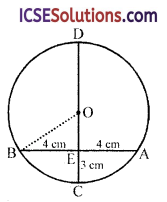Solution:
In the figure,
CD is the diameter of the circle with centre O
AB is chord which intersects CD at E and EA = EB = 4 cm and CE = 3 cm
Let r be the radius of the circle then
OC = r and OE = (r – 3)
Join OB In right ∆OBC,
OB² = BE² + OE² (Pythagoras Theorem)
⇒ r² = (4)² + (r – 3)²
⇒ r² = 16 + r² – 6r + 9
⇒ r² – r² + 6r = 16 + 9
⇒ 6r = 25 ⇒ r = $$\frac { 25 }{ 6 }$$ = 4$$\frac { 1 }{ 6 }$$
∴ Radius of the circle = 4$$\frac { 1 }{ 6 }$$ cm

Question 4.
In a circle of radius 5 cm, AB and CD are two parallel chords of length 8 cm and 6 cm respectively. Calculate the distance between the chords, if they are on
(i) the same side of the centre ;
(ii) opposite sides of the centre.
Solution:
(i) When the chords are in the same side of the centre
Radius of the circle = 5 cm
Length of AB = 8 cm and CD = 6 cm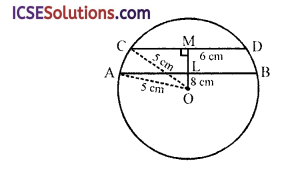From O, draw a perpendicular on CD which intersects AB at L and meets CD at M
∴ M is the midpoints of CD and L is the midpoint ofAB
Join OA and OC
Now in right ∆OCM,
OC² = CM² + OM² (Pythagoras Theorem)
⇒ (5)² = (3)² + OM² (∵ CM = $$\frac { 1 }{ 2 }$$ CD = $$\frac { 1 }{ 2 }$$ x 6 = 3 cm)
⇒ 25 = 9 + OM² ⇒ OM² = 25 – 9 = 16 = (4)²
∴ OM = 4 cm
Similarly in right angled ∆OAL,
OA² = AL² + OL²
⇒ (5)² = (4)² + OL² (AL = $$\frac { 1 }{ 2 }$$ AB = $$\frac { 1 }{ 2 }$$ x 8 = 4 cm)
25 = 16 + OL²
⇒ OL² = 25 – 16 = 9 = (3)²
∴ OL = 3 cm
Now LM = OM – OL = 4 – 3 = 1 cm
∴ Distance between the two chords = 1 cm

(ii) When the chords are in the opposite sides of the centre
Draw OL ⊥ AB and OM ⊥ CD
In right ∆OCM,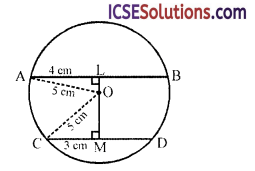OC² = CM² + OM² (Pythagoras Theorem)
⇒ (5)² = (3)² + OM² (∵ CM = $$\frac { 1 }{ 2 }$$ CD)
⇒ 25 = 9 + OM²
⇒ OM² = 25 – 9 = 16 = (4)²
OM = 4 cm
Similarly in right ∆OLA,
OA² = OL² + AL²
⇒ (5)² = OL² + (4)² (∵ AL = $$\frac { 1 }{ 2 }$$ AB)
⇒ 25 = OL² + 16
OL² = 25 – 16 = 9 = (3)²
∴ OL = 3 cm
∴ LM = OL + OM = 3 + 4 = 7 cm
∴ Distance between the two chords = 7 cmQuestion 5.
The radius of a circle is 2.5 cm. AB, CF are two parallel chords 3.9 cm apart. If AB = 1.4 cm, find CF.
Solution:
In right angled ∆OAM,
AM = 0.7 cm
OA = 2.5 cm and OA² = AM² + OM²
(Pythagoras Theorem)⇒ (2.5)² = (0.7)² + OM²
⇒ 6.25 = 0.49 + OM²
⇒ OM² = 6.25 – 0.49 = 5.76 = (2.4)²
∴ OM = 2.4
But LM = 3.9
∴ OL = LM – OM = 3.9 – 2.4 = 1.5 cm
Now in ∆OLC,
OC² = CL² + OL² (Pythagoras Theorem)
⇒ (2.5)² = CL² + (1.5)²
⇒ 6.25 = CL² + 2.25 CL²
= 6.25 – 2.25 = 4.00 = (2)²
∴ CL = 2
∴ Chord CF = 2 x CL = 2 x 2 = 4 cm

Question 6.
A chord distant 2 cm from the centre of a circle is 18 cm long. Calculate the length of a chord of the same circle which is 6 cm distant from the centre.
Solution:
Let r be the radius of the circle and chord
AB = 18 cm
and distance from the centre O
∴ OL = 2 cm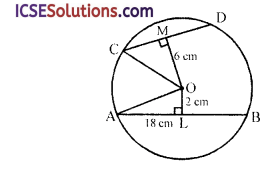Let CD be another chord
OM ⊥ CD and OM = 6 cm
Now in right ∆OAL,
OA² = AL² + OL² (Pythagoras Theorem)
= (9)² + (2)² = 81 + 4 = 85 (AL = $$\frac { 1 }{ 2 }$$AB = $$\frac { 1 }{ 2 }$$ x 18 = 9 cm)
Similarly in right ∆OCM,
OC² = OM² + CM²
⇒ OA² = OM² + CM² (∵ OA = OC radii of the same circle)
⇒ 85 = (6)² + CM²
⇒ 85 = 36 + CM² ⇒ CM² = 85 – 36 = 49 = (7)²
∴ CM = 7
∴ CD = 2 x CM
= 2 x 7 = 14cm

Question 7.
In figure, circles are concentric with centre O. Find AC.Solution:
In the figure, two circles are concentric with centre O
Chord AB intersect the small circle at C and D
OB and OC are joined
OB = 17 cm, OC = 10 cm
OL ⊥ AB
Now in right ∆OMB,
OB2 = OM² + MB² (Pythagoras Theorem)
⇒ (17)² = (9)² + MB²
⇒ 289 = 81 + MB²
⇒ MB² = 289 – 81 = 208
MB = $$\sqrt{208}$$ = 14.42
or AM = 14.42 cm (∵ M is midpoint of AB)
Similarly in right ∆OCM,
OC² = OM² + CM²
⇒ (10)² = (9)² + CM²
⇒ 100 = 81 + CM²
⇒ CM² = 100 – 81 = 19
∴ CM = $$\sqrt{19}$$ = 4.36 cm
∴ AC = AM – CM = 14.42 – 4.36 = 10.06 cm

Question 8.
The length of the common chord of two equal intersecting circles is 10 cm and the distance between the two centres is 6 cm. Find the radius of each circle.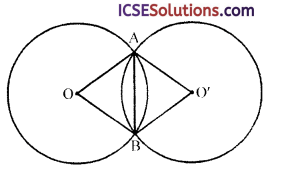Solution:
Two equal circles with centre O and O’ intersect each other at A and B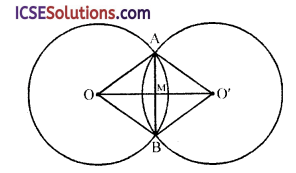OO’, AB, OA, OB, O’A and O’B are joined
AB = 10 cm
OO’ = 6 cm
Let OO’ bisects AB at M i.e. AM = MB = $$\frac { 10 }{ 2 }$$ = 5cm
and OM = MO’ = $$\frac { 6 }{ 2 }$$ = 3 cm
Now in right ∆OAM,
OA² = OM² + AM² (Pythagoras Theorem)
= (3)² + (5)² = 9 + 25 = 34
∴ OA = $$\sqrt{34}$$ = 5.83 cm
Hence radius of each circle = 5.83 cm

Question 9.
In figure, AB = 8 cm, CM = 1 cm, CM is the perpendicular bisector of AB. The radius OA = x cm. Find x.Solution:
In circle with centre O, OA is its radius
AB is chord, OM ⊥ AB which is produced to meet the circle at C
AB = 8 cm, CM = 1 cm, OA = x
OC = OA = x
∴ OM = OC – CM = (x – 1)
Now in right ∆OAM,
OA² = AM² + OM² (Pythagoras Theorem)
x² = (4)² + (x – 1)² (AM = $$\frac { 1 }{ 2 }$$ AB = $$\frac { 1 }{ 2 }$$ x 8 = 4 cm)
⇒ x² = 16 + x² – 2x + 1
⇒ x² – x² + 2x = 17
⇒ 2x = 17 ⇒ x = $$\frac { 17 }{ 2 }$$ = 8.5
Hence x = 8.5 cm

Question 10.
In figure, CD is the perpendicular bisector of the chord AB. If AB = 2 cm and CD = 4 cm, calculate the radius of the circle.Solution:
In the circle with centre O, AB is chord CD is perpendicular bisector of ABAB = 2 cm, CD = 4 cm
Join OA, let r be the radius of the circle
OA = OD = r
∴ OC = CD – OD = 4 – r
AC = $$\frac { 1 }{ 2 }$$ AB = $$\frac { 1 }{ 2 }$$ x 2 = 1 cm
Now in right ∆OAC,
OA² = OC² + AC² (Pythagoras Theorem)
⇒ r² = (4 – r)² + (1)²
⇒ r² = 16 + r² – 8r + 1
⇒ r² – r² + 8r = 17
⇒ 8r = 17
⇒ r = $$\frac { 17 }{ 8 }$$ = 2$$\frac { 1 }{ 8 }$$
∴ Radius of the circle = 2 $$\frac { 1 }{ 8 }$$ cm

Question 11.
In figure, OD is perpendicular to the chord AB of a circle, whose centre is O. Prove that CA = 2OD.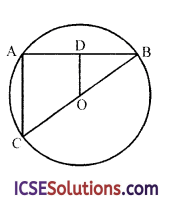Solution:
Given : In the circle AB is chord, OD ⊥ AB
BOC is the diameter of the circle
AC is joined
To prove : CA = 2OD
Proof: OD ⊥ AB
∴ D is midpoint of AB
and O is midpoint of BC
In ∆ABC, DO || AC
∴ In ∆ABC ~ ∆DBO,
∴ $$\frac{\mathrm{AB}}{\mathrm{BD}}=\frac{\mathrm{BC}}{\mathrm{BO}}=\frac{\mathrm{AC}}{\mathrm{OD}}$$
(Sides of similar triangles are proportional)
⇒ $$\frac{\mathrm{AB}}{\frac{1}{2} \mathrm{AB}}=\frac{\mathrm{CA}}{\mathrm{OD}} \Rightarrow \frac{2}{1}=\frac{\mathrm{CA}}{\mathrm{OD}}$$ ⇒ CA = 2OD
Hence proved.

Question 12.
In figure, OMNP is a square. A circle drawn with centre O cuts the square in X and Y. Prove that : ∆OXM ≅ ∆OYP. Hence prove that NX = NY.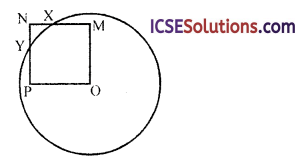Solution:
Given : In the figure,
OMNP is a square
A circle with centre O is drawn which intersects the square at X and Y
Join OX and OY(i) ∆OXM ≅ ∆OYP
(ii) NX = NY
Proof: In right ∆OXM and ∆OYP,
Hyp. OX = OY (radii of the same circle)
Side OM = OP (sides of a square)
∴ ∆OXM ≅ ∆OYP (RHS axiom)
∴ MX = PY
But MN = PN (sides of a square)
∴ MN – MX = PN – PY
⇒ NX = NY
Hence proved.
∴ Least value of x = 2 cm and greatest = 8 cmQuestion 13.
Find the centre of given circle.
Solution:
Steps of construction :
(i) Take three points A, B and C on the circle.
(ii) Join AB and AC.
(iii) Draw the perpendicular bisectors of AB and AC. Which intersect each other at O.
Then O is the centre of the circle
(We know that the perpendicular bisectors of chords of a circle passes through the centre of the circle) [Hint, see fig. 15.36]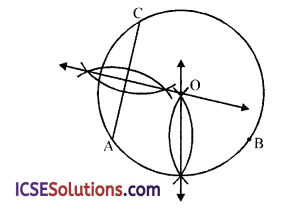Question 14.
Draw an arc of a circle to pass through three points A, B and C not in the same st. line.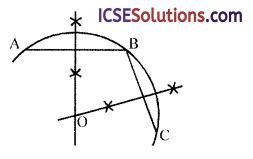Solution:
Steps of construction :
(i) Take three points A, B and C.
(ii) Join AB and BC.
(iii) Draw the perpendicular bisector of AB and BC intersecting each other at O.
(iv) With centre O and radius OA or OB or OC, draw an arc ABC.
This is the required arc.
∵ O lies on the perpendicular bisector of AB.
∴ OA = OB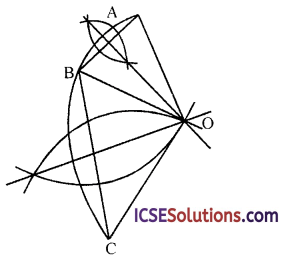Again O lies on the perpendicular bisector of BC
∴ OB = OC
∴ OA = OB = OC

Question 15.
Answer true or false. Three circles can pass through three given points not in a straight line.
Solution:
False : as through three points which are not in a straight line, one and only one circle can be drawn.

Question 16.
Two chords A B and CD of a circle are parallel and a line l is the perpendicular bisector of AB. Show that l bisects CD.
Solution:
AB and CD are two parallel chords of the circle and a line / is the perpendicular bisector of AB
i. e., AL = LB and ∠ALM = 90°To prove : Line l is also perpendicular bisector of CD
Proof: ∵ Line l is perpendicular bisector of chord AB
∴ It will pass through the centre of the circle
∵ AB || CD and l is perpendicular to AB
∴ l is also perpendicular to CD
∵ OM ⊥ CD
∴ M is the midpoint of CD
∴ l bisects CD
Hence proved.

Question 17.
In the given figure, O and O’ are the centres of two intersecting circles and APB is parallel to 00′. Prove that AB = 200′.Solution:
Given : Two circles with centre O and O’
intersect each other at P
APB || OO’ is drawn
To prove : AB = 200′
Construction : From O, draw OM ⊥ AP
and from O’, O’N ⊥ PB
Proof: ∵ OM ⊥ AP and O’N ⊥ PM
and OO’ || AB
∴ MN = OO’
Now ∵ OM ⊥ AP
∴ M is midpoint of AP
AM = MP ⇒ MP = $$\frac { 1 }{ 2 }$$ AP
Similarly PN = NB ⇒ PN = $$\frac { 1 }{ 2 }$$ PB
MP + PN = $$\frac { 1 }{ 2 }$$ AP + $$\frac { 1 }{ 2 }$$ PB
⇒ MN = $$\frac { 1 }{ 2 }$$ (AP + PB) = $$\frac { 1 }{ 2 }$$ AB
⇒ OO’ = $$\frac { 1 }{ 2 }$$ AB (∵ OO’ = MN)
⇒ 200′ = AB
or AB = 200′
Hence proved.

Question 18.
Two equal chords AB and CD of a circle intersect at P, show that AP = PD and BP = CP.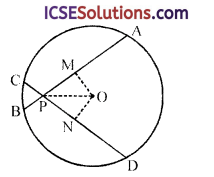Solution:
Given : In a circle with centre O,
Two equal chords AB and CD intersect each other at P
To prove : AP = PD and BP = CP
Construction : Draw OM ⊥ AB and ON ⊥ CD
Join OP
Proof : In right ∆OMP and ∆ONP,
Hyp. OP = OP (common)
Side OM = ON
(Equal chords are equidistant from the centre)
∴ ∆OMP ≅ ∆ONP (R.H.S. axiom)
∴ PM = PN (c.p.c.t.)
∵ OM ⊥ AB and ON ⊥ CD
∴ M and N are the midpoints of AB and CD respectively
or AM = MP and CN = NP
∵ Chords AB = CD
∴ AB – PB = CD – PC
⇒ AP = PD
Similarly BM – PM = CN – PN (∵ PM = PN)
⇒ BP = CP
Hence proved.Question 19.
In the figure, shows circle with centre O, with equal chords AB and CD; OE ⊥CD at H and OF ⊥ AB at G. Prove that EH = GF.Solution:
Given : In a circle with centre O,
chord AB = CD
OE ⊥ CD at A and OF ⊥ AB at G
To prove : EH = GF
Proof: ∵ OE ⊥ CD and OF ⊥ AB
∴ G and H are midpoints of AB and CD respectively
∵ and OG = OH
(Equal chords are equidistant from the centre)
But OF = OE (radii of the same circle)
∴ OF – OG = OE – OH
⇒ GF = EH
∴ EH = GF
Hence proved.

Question 20.
In the figure, C is the centre of the circle. CB bisects the ∠DBE, CD ⊥ PQ and CE ⊥ RS. Prove that PQ = RS.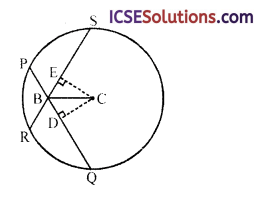Solution:
Given : In circle with centre C,
CB bisects ∠DBE
CD ⊥ PQ and CE ⊥ RS where PQ and RS are the chords which intersect each other at B
To prove : PQ = RS
Proof: In ∆EBC and ∆DBC,
∠E = ∠D (each 90°)
∠EBC = ∠DBC
(∵ BC is the bisector of ∠DBE)
BC = BC (common)
∴ ∆EBC ≅ ∆DBC (AAS axiom)
∴ and CE = CD (c.p.c.t.)
But these are the perpendicular distance from the centre and equal chords are equidistant from the centre
∴ RS = PQ
or PQ = RS
Hence proved.

Question 21.
In the figure, AB and CD are equal chords of a circle whose centre is O. OM ⊥ AB and ON ⊥ CD. Prove that ∠OMN = ∠ONM.Solution:
Given : In circle with centre O,
Two equal chords AB and CD intersect each other inside the circle
OM ⊥ AB and ON ⊥ CD
MN arejoined
To prove : ∠OMN = ∠ONM
Proof: ∵ Chord AB = CD
∴ OM = ON
(Equal chords are equidistant from the centre)
In ∆OMN,
∵ OM = ON
∴ ∠OMN = ∠ONM
Hence proved.